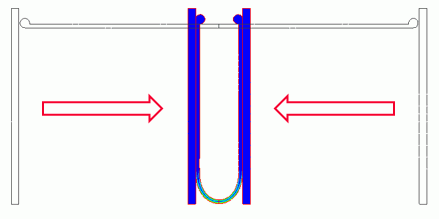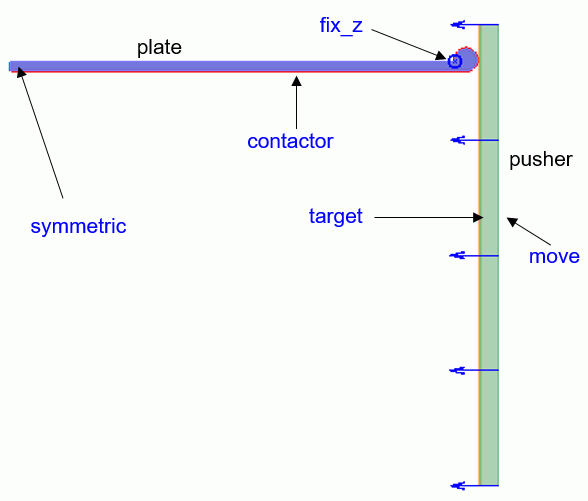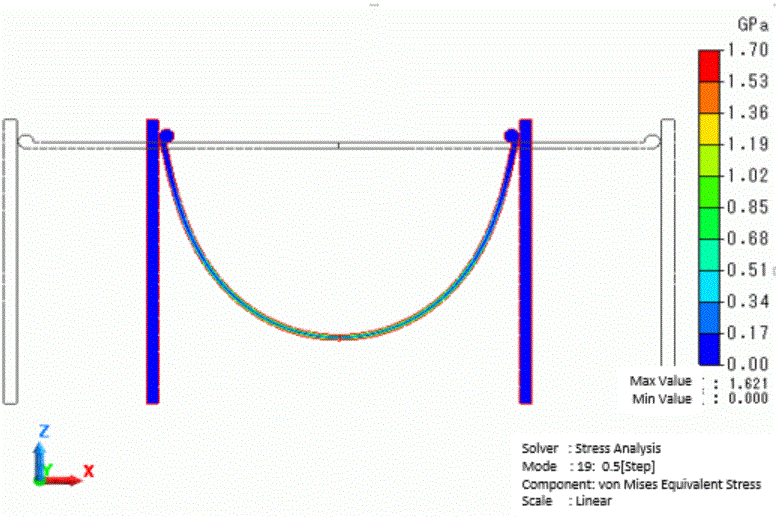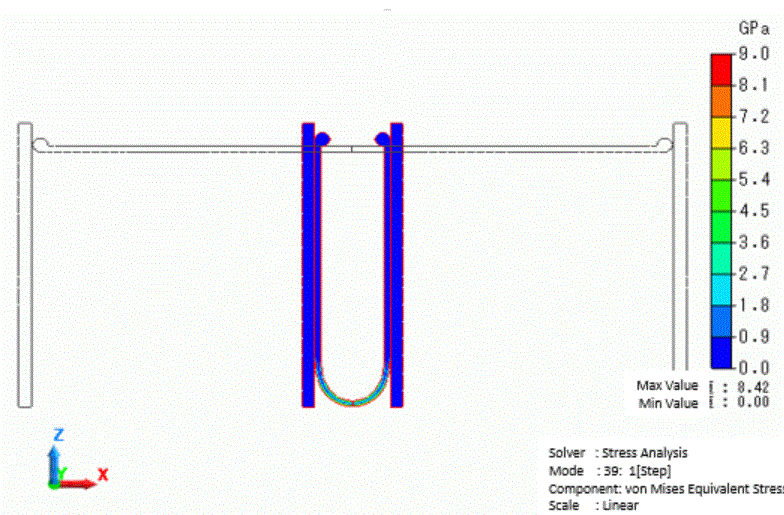﻿ Contact Analysis of Bending PlateExamples | Product | Murata Software Co., Ltd.Example65Contact Analysis of Bending PlateGeneral

• This is an example of a contact analysis of the process of bending a plate into a U-shape by pressing it with other plates from both sides.

• The deformation, the displacement distribution, and the stress distribution are solved.

• Unless specified in the list below, the default conditions will be applied.

Analysis Space

 Item Setting Analysis Space 2D Model unit mm

Analysis Condition

“Large Deformation” is selected as the analysis involves the large deformation with rotation.

 Item Setting Solver Stress analysis [Galileo] Analysis Type Static Analysis Options Select Large Deformation

The Step/Thermal Load is set as follows.

Since the deformation is large, the number of substeps is increased to ensure the convergence of calculation.

 Tab Setting Item Setting Step/Thermal Load Step/Reached Temperature Setting Number of Substeps : 40 Options for the nonlinear analysis Select Save the results of substeps

Model

A symmetric boundary (symmetric) and a contactor face (contactor) are set to the plate that is to be bent.

Also, a fixed Z-displacement boundary (fix_z) is set for the stable bending.The contacting parts are made circular so that the plate bends downward convexly.

The circular form is adjusted so that the initial contact occurs above the fixed Z-displacement boundary (fix_z).

A contactee surface (target) and a displacement boundary (move) are set to the plate that is used for bending the other plate.Body Attributes and Materials

 Body Number/Type Body Attribute Name Material Name 1/Sheet pusher 007_Fe * 3/Sheet plate 001_Al *

* Available from the material DB

Boundary Condition

The boundary conditions are set as follows.

 Boundary Condition Name/Topology Tab Boundary Condition Type Setting fix_z/vertex Mechanical Displacement Select the Z Component. UX=0 Move/Edge Mechanical Displacement Select the X and Z components. UX= -45 x 10-3 [m] UZ= 0.0 x 10-3 [m] contactor/Edge Mechanical Contact Surface Contactor surface target/Edge Mechanical Contact Surface Contactee surface Symmetric/Edge Symmetry/Continuity Symmetry Select Reflective

The contact pair is set as below. The coefficient of friction is set to 0.

 Boundary Pair Coefficient of Friction contactor-target 0.0

Results

The displacement and the von Mises stress distribution at the step 0.5 are shown below.The stresses are exhibited over the entire plate body.

Below are the displacement and the von Mises stress distribution at the step 1.0.Since the bending deformation of the body is concentrated in the center part, the mechanical stress also occurs in the same part.

﻿